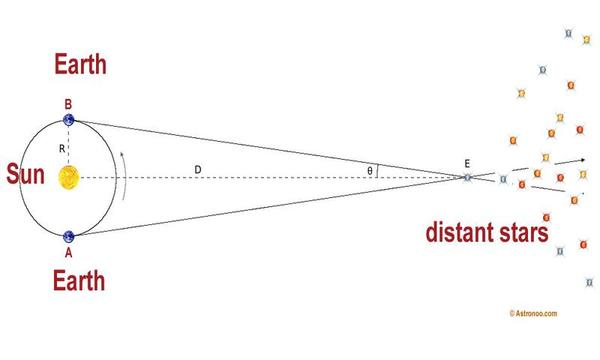# How to measure distances in the Universe?Image: The annual parallax is used to assess the distance of a star. Scientists measure the parallax angle by recording the position of a star on the celestial vault, at 6 month intervals. To do this, just take measuring from a position of the Earth in its orbit, and measuring again 6 months later when the Earth is on the other side of the Sun. The diameter of the Earth's orbit is ≈300 million km, the parallax angle gives the distance by a simple trigonometric calculation.

### Methods: parallax, standard candles, supernova

You probably know that the Universe is very big, and there are many different things in it. To measure distances in the Universe, scientists use different methods depending on the distance they are trying to measure.

For closer distances, such as those between stars in our galaxy, scientists use a method called parallax. This involves measuring the position of the star at different times of the year as the Earth moves around the Sun. Using these measurements, scientists can determine the star's distance using mathematical calculations.

For farther distances, scientists use light from supernovae, which are star explosions that produce an incredible amount of light like a billion sun. By measuring the brightness of the supernova, scientists can determine its distance.

Another method is to use the relationship between size and luminosity of stars called standard candles. This relationship allows scientists to determine the distance of variable stars, which are stars that change brightness on a regular basis.

Finally, for even further distances, scientists use light from quasars, which are very bright and distant objects. By measuring the light from quasars, scientists can determine their distance.

1997 © Astronoo.com − Astronomy, Astrophysics, Evolution and Earth science.